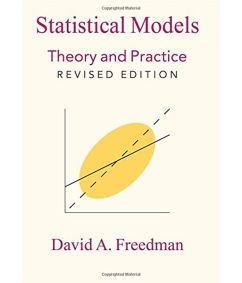Monday , December 4 2023Home / Lars P. Syll / Resisting the ‘statistical significance testing’ temptation

# Resisting the ‘statistical significance testing’ temptationby
Lars Pålsson Syll
My articles My siteAbout meMy booksMy videos
Summary:
Resisting the ‘statistical significance testing’ temptation Imagine a dictator “game” in which a mixed-sex group of experimental subjects are used as first players who can decide which share of their initial endowment they give to a second player (one person acts as second player for the whole group). Additionally, assume that the experimental subjects are a convenience sample but not a random sample of a well-defined broader population. What kind of statistical inferences are possible? Neither one of the two chance mechanisms – neither random sampling nor randomization –  applies. Consequently, there is no role for the p-value … Due to engrained disciplinary habits, researchers might be tempted to implement “statistical significance testing” routines in

Topics:
Lars Pålsson Syll considers the following as important:

This could be interesting, too:

Lars Pålsson Syll writes Improving econometric education

Lars Pålsson Syll writes Why we need causality in science

Lars Pålsson Syll writes Improving econometric analysis

Lars Pålsson Syll writes Guido Imbens on the response to LATE

## Resisting the ‘statistical significance testing’ temptation

Imagine a dictator “game” in which a mixed-sex group of experimental subjects are used as first players who can decide which share of their initial endowment they give to a second player (one person acts as second player for the whole group). Additionally, assume that the experimental subjects are a convenience sample but not a random sample of a well-defined broader population. What kind of statistical inferences are possible? Neither one of the two chance mechanisms – neither random sampling nor randomization –  applies. Consequently, there is no role for the p-value …Due to engrained disciplinary habits, researchers might be tempted to implement “statistical significance testing” routines in our dictator game example even though there is no chance model upon which to base statistical inference.  While there is no random process, implementing a two-sample t-test might be the spontaneous reflex to find out whether there is a “statistically significant” difference between the two sexes. One should recognize, however, that doing so would require that some notion of a random mechanism is accepted. In our case, this would require imagining a randomization distribution that would result if money amounts were repeatedly assigned to sexes (“treatments”) at random. Our question would be whether the money amounts transferred to the second player differed more between the sexes than what would be expected in the case of such a random assignment. We must realize, however, that there was no random assignment of subjects to treatments, i.e. the sexes might not be independent of covariates. Therefore, the p-value based on a two-sample t-test for a difference in mean does not address the question of whether the difference in the average transferred money is caused by the subjects’ being male or female. That could be the case, but the difference could also be due to other reasons such as female subjects being less or more wealthy than male subjects. As stated above, it would therefore make sense to control for known confounders in a regression analysis ex post – again, without reference to a p-value as long as the experimental subjects have not been recruited through random sampling.

Norbert Hirschauer et al.As shown over and over again when significance tests are applied, people have a tendency to read ‘not disconfirmed’ as ‘probably confirmed.’ Standard scientific methodology tells us that when there is only say a 10 % probability that pure sampling error could account for the observed difference between the data and the null hypothesis, it would be more ‘reasonable’ to conclude that we have a case of disconfirmation. Especially if we perform many independent tests of our hypothesis and they all give about the same 10 % result as our reported one, I guess most researchers would count the hypothesis as even more disconfirmed.

We should never forget that the underlying parameters we use when performing significance tests are model constructions. Our p-values mean nothing if the model is wrong. And most importantly — statistical significance tests DO NOT validate models!

In journal articles, a typical regression equation will have an intercept and several explanatory variables. The regression output will usually include an F-test, with p – 1 degrees of freedom in the numerator and n – p in the denominator. The null hypothesis will not be stated. The missing null hypothesis is that all the coefficients vanish, except the intercept.If F is significant, that is often thought to validate the model. Mistake. The F-test takes the model as given. Significance only means this: if the model is right and the coefficients are 0, it is very unlikely to get such a big F-statistic. Logically, there are three possibilities on the table:
i) An unlikely event occurred.
ii) Or the model is right and some of the coefficients differ from 0.
iii) Or the model is wrong.

So?Professor at Malmö University. Primary research interest - the philosophy, history and methodology of economics.